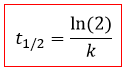# Problem: Fluorine-18 is a radioactive isotope that decays by positron emission to form oxygen-18 with a half-life of 109.7 min. (A positron is a particle with the mass of an electron and a single unit of positive charge; the equation is 9 18F⟶ 8 18O + +10e)Physicians use 18F to study the brain by injecting a quantity of fluoro-substituted glucose into the blood of a patient. The glucose accumulates in the regions where the brain is active and needs nourishment.(b) If a sample of glucose containing radioactive fluorine-18 is injected into the blood, what percent of the radioactivity will remain after 5.59 h?

🤓 Based on our data, we think this question is relevant for Professor Briggs' class at ASU.

###### FREE Expert Solution

We can determine [A]t, but lack the value of k.

can be obtained from the equation of half-life for 1st order reactions:Step 1: We rearrange the half-life equation to solve for k :

$\overline{){\mathbf{k}}{\mathbf{=}}\frac{\mathbf{ln}\left(2\right)}{{\mathbf{t}}_{\mathbf{1}}{\mathbf{2}}}}}$

6.32×10-3 min-1

Step 2: We convert the time elapsed to minutes:

335.4 min

Step 3: We determine [A]t in the 1st Order Rate Law:

ln [A]t = -2.119728

Ln has a base of e. When you divide a number by ln you will take e to the power of the number.###### Problem Details

Fluorine-18 is a radioactive isotope that decays by positron emission to form oxygen-18 with a half-life of 109.7 min. (A positron is a particle with the mass of an electron and a single unit of positive charge; the equation is 9 18F⟶ 8 18O + +10e)
Physicians use 18F to study the brain by injecting a quantity of fluoro-substituted glucose into the blood of a patient. The glucose accumulates in the regions where the brain is active and needs nourishment.

(b) If a sample of glucose containing radioactive fluorine-18 is injected into the blood, what percent of the radioactivity will remain after 5.59 h?